# Chapter 4 Quadrilateral Equations NCERT Exemplar Solutions Exercise 4.1 Class 10 Maths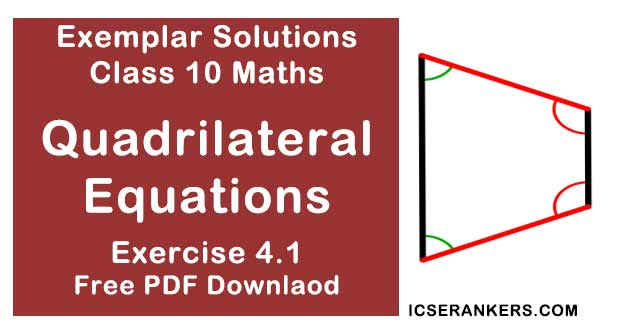Chapter Name NCERT Maths Exemplar Solutions for Chapter 4 Quadrilateral Equations Exercise 4.1 Book Name NCERT Exemplar for Class 10 Maths Other Exercises Exercise 4.2Exercise 4.3Exercise 4.4 Related Study NCERT Solutions for Class 10 Maths

### Exercise 4.1 Solutions

Choose the correct answer from the given four options in the following questions:

1. Which of the following is a quadratic equation?
(A) x2 +2x + 1 = (4 – x)2 + 3
(B) -2x2 = (5 – x)(2x – 2/5)
(C) (k + 1)x2 + (3/2)x = 7, where k = -1
(D) x3 - x2 = (x - 1)3

Solution

Correct answer is (D) x3 - x2 = (x - 1)3
Justification :
ax2 + bx + c = 0,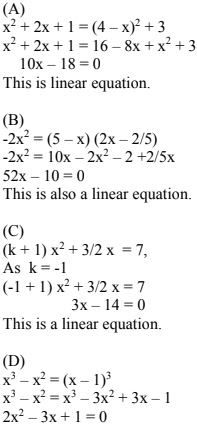Above equation represents a quadratic equation.

2. Which of the following is not a quadratic equation ?
(A) 2(x - 1)2 = 4x2 - 2x + 1
(B) 2x - x2 = x2  + 5
(C) (√2x + √3)2 + x2 = 3x2 - 5x
(D) (x2 + 2x)2 = x4 + 3 + 4x3

Solution

Correct answer is (D) (x2 + 2x)2 = x4 + 3 + 4x3
Equation to be quadratic, it should be in the form,
ax2 + bx + c = 0, a ≠ 0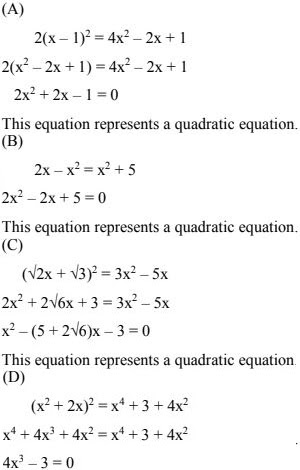This equation represents a cubic equation.

3. Which of the following equation has  2 as a root ?
(A) x2 - 4x + 5 = 0
(B) x2 + 3x - 12 = 0
(C) 2x2 - 7x + 6 = 0
(D) 3x2 - 6x - 2 = 0

Solution

Correct answer is (C) 2x2 - 7x + 6 = 0
As 2 is a root then putting value 2 in place of x, we should get zero.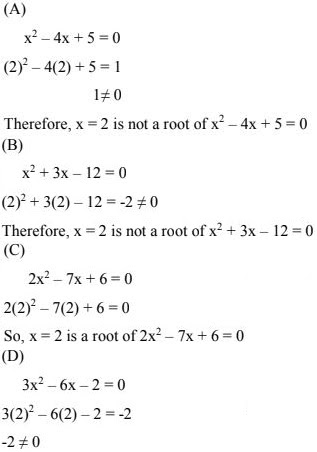Therefore, x = 2 is not a root of 3x2 - 6x - 2 = 0

4. If 1/2 is a root of the equation x2 + kx - 5/4 = 0 , then the value of k is
(A) 2
(B) -2
(C) 1/4
(D) 1/2

Solution

As, 1/2 is a root of the equation x2 + kx - 5/4 = 0.
Putting the value of 1/2 in place of x gives us the value of k.
As,
x = 1/2
(1/2)2 + k(1/2) - (5/4) = 0
⇒ (k/2) = (5/4) - 1/4
So, k = 2

5. Which of the following equations has the sum of its roots as 3 ?
(A) 2x2 - 3x + 6 = 0
(B) -x2 + 3x - 3 = 0
(C) √2x2 - 3/√2x + 1 = 0
(D) 3x2 - 3x + 3 = 0

Solution

Correct answer is (B) -x2 + 3x - 3 = 0.
The sum of the roots of a quadratic equation ax2 + bx + c = 0, a ≠ 0 is given by,
Coefficient of x/coefficient of x2 = -(b/a)
(A) We have,
2x2 - 3x + 6 = 0
Sum of the roots = -b/a
= - (-3/2)
Sum of the roots = 3/2
(B) We have,
-x2 + 3x - 3 = 0
Sum of the roots = -b/a
= -(3/-1) = 3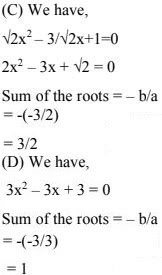6. Value(s) of k for which the quadratic equation 2x2 – kx + k = 0 has equal roots is
(a) 0 only
(b) 4
(c) 8 only
(d) 0, 8

Solution

(D) The condition for equal roots of quadratic equation ax2 + bx + c = 0 is
b2 - 4ac = 0.

We have,
2x2 - kx + k = 0
Condition for equal roots,
b2 - 4ac = 0
⇒ (-k)2 - 4(2)(k) = 0
⇒ k2 - 8k = 0
⇒ k(k - 8) = 0
⇒ k = 0
Or
k - 8 = 0
⇒ k = 8
As, the values of k are 0 and 8.

7. Which constant must be added and subtracted to solve the quadratic equation
9x2 + 3x/4 - √2 = 0
by the method of completing the square ?
(a) 1/8
(b) 1/64
(c) 1/4
(d) 9/64

Solution

(b) 1/64
The given equation is
9x2 + 3x/4 - √2 = 0
So, to make the expression a complete square, we have to subtract 1/64.
9x2 + 3x/4 + 1/64 - √2 - 1/64 = 0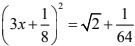8. The quadratic equation has :
2x2 - √5x + 1 = 0
(A) two distinct real roots
(B) two equal real roots
(C) no real roots
(D) more than two real roots

Solution

(C) no real roots
We have,
2x2 - √5x + 1 = 0
Now,
D = b2 - 4ac,
Checking the following conditions:
(i) for no real roots D < 0
(ii) for two equal roots, D = 0
(iii) for two distinct roots D > 0 and any quadratic equation must have only roots.
The equation is :
2x2 - √5x + 1 = 0
So,
D = b2 - 4ac
Where,
a = 2 ,
b = -√5
c = 1
D = 5 - 8
⇒ D = - 3
As D < 0 So, the given equations has no real roots.

9. Which of the following equations has two distinct real roots ?
(a) 2x2 - 3√2x + 9/4 = 0
(b) x2 + x - 5 = 0
(c) x2 + 3x + 2√2 = 0
(d) 5x2 - 3x + 1 = 0
Solution

Correct answer is (b) x2 + x - 5 = 0
We have,
For real distinct roots D > 0
(A) Equation is 2x2 - 3√2x + 9/4 = 0
D = b2 - 4ac
⇒ D = 9 × 2 - 18
⇒ D = 0
For, D = 0, the given equation has two real equal roots.

(B) Equation is x2 + x - 5 = 0
D = b2 - 4ac
⇒ D = (1)2 - 4(1)(-5)  (where, a = 1, b = 1, c = -5)
⇒ D = 1 + 20
⇒ D = 21
For, D > 0, the given equation has two distinct real roots.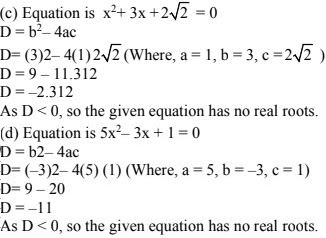10. Which of the following equations has no real roots.
(a) x2 - 4x + 3√2 = 0
(b) x2 + 4x - 3√2 = 0
(c) x2 - 4x - 3√2 = 0
(d) 3x2 + 4√3x + 4 = 0
Solution

Correct answer is (a) x2 - 4x + 3√2 = 0
Given equation is x2 - 4x + 3√2 = 0
D = b2 - 4ac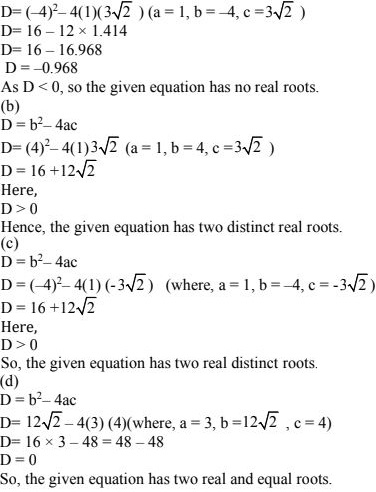11. (x2 + 1)2 - x2 = 0 has
(a) four real roots
(b) two real roots
(c) no real roots
(d) one real root

Solution

(c) no real roots
We have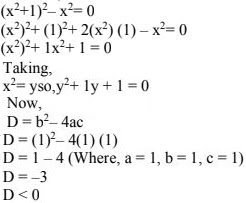So, the given equation y2 + y + 1 = 0 has no values of y in equation y2 + 1y + 1 = 0 or if y is not real then x2 will not be real so no values of x are real or the given equation has no real roots.